# Remove Duplicate Elements from Sorted Array

July 19, 2020 24113

Duplicates are always adjacent in a sorted array. For example, in the array {1,3,5,5,7,9}, 5 is a duplicate element. Let’s write program to remove duplicate elements.

## Method 1 – Using extra space

Suppose we want to remove duplicate elements from an array arr. For that, we will run a for loop in which i will point to the index of each element of arr. We will make another pointer j that will point to the index of the elements of a new array temp where non-duplicate elements will be stored. We will initialize j by 0 as temp is initially empty. Then we will check whether the ith element of arr is equal to the (i+1)th element .

If it is, then

-- We will increment i by 1

else

-- We will store the ith element in temp. Then we will increment i by 1 and j by 1.

We will run the for loop from the 1st element till the second last element of arr. We will not include the last element in the for loop as there is no (i+1)th element for the last element. After the loop is completed, store the last element in temp and increment j.

Then copy the j elements of temp to arr and print the same.

### Example

Let us take an array arr[] ={1,3,5,5,7,9}. In the first iteration of the for loop, i will point to the index of the first element (1). Then we will check if the ith element is equal to the (i+1)th element.

1 is not equal to 3, so the ith element (1) will be stored in another array temp and i will be incremented by 1 so that it points to the index of the second element (3).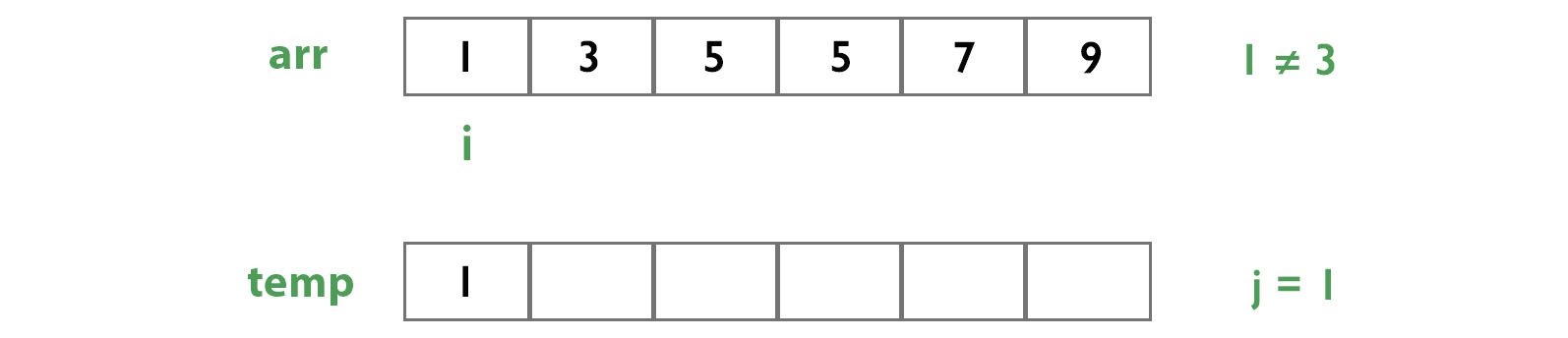Again, we will check whether 3 is equal to 5. It is not equal, so 3 will be stored in temp and i will be incremented by 1 so that it points to the index of the third element (5).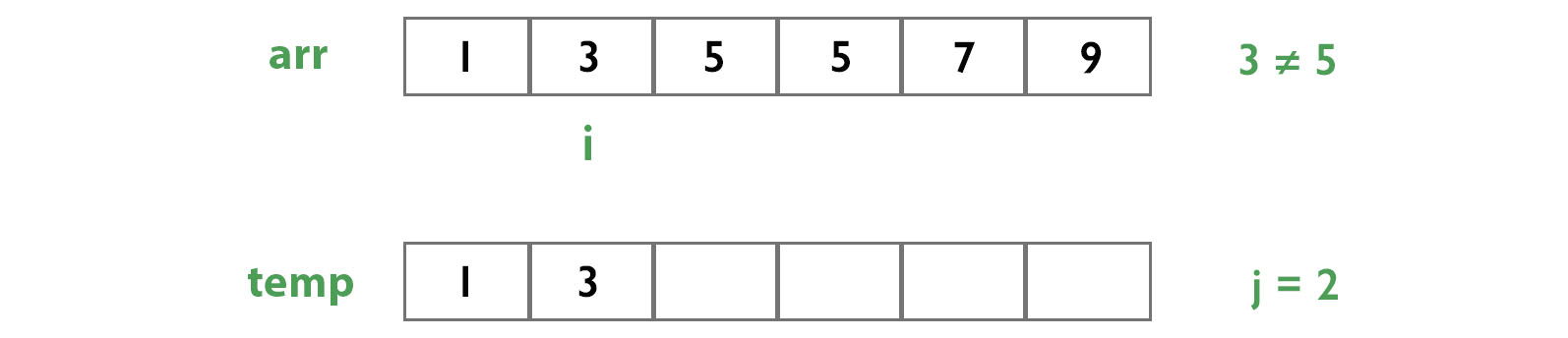This time, the ith element (5) is equal to the (i+1)th element (5). So, the ith element will not be stored in temp and i will be incremented so that it points to the index of the next 5.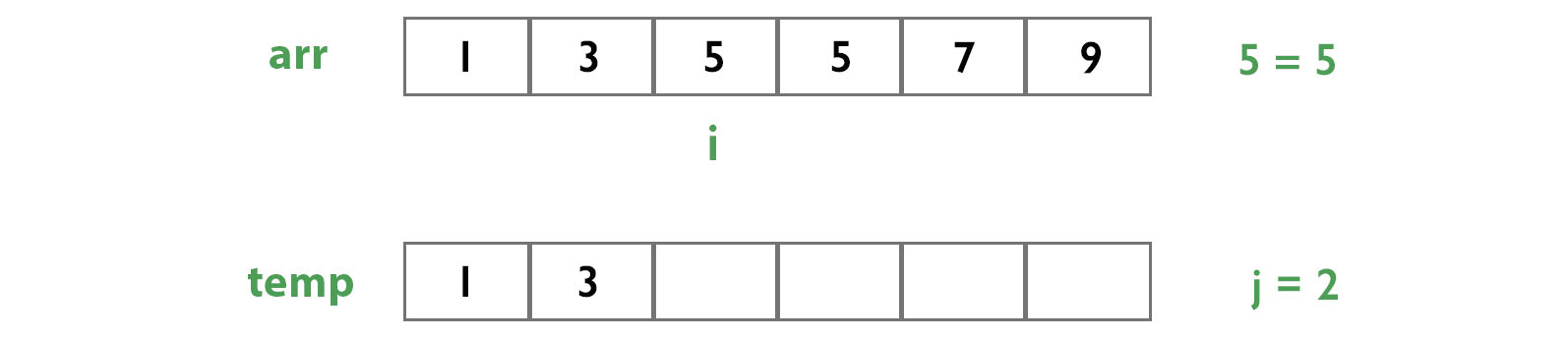Again, 5 is not equal to 7. So, 5 will be stored in temp and i will be incremented so that it points to the index of 7.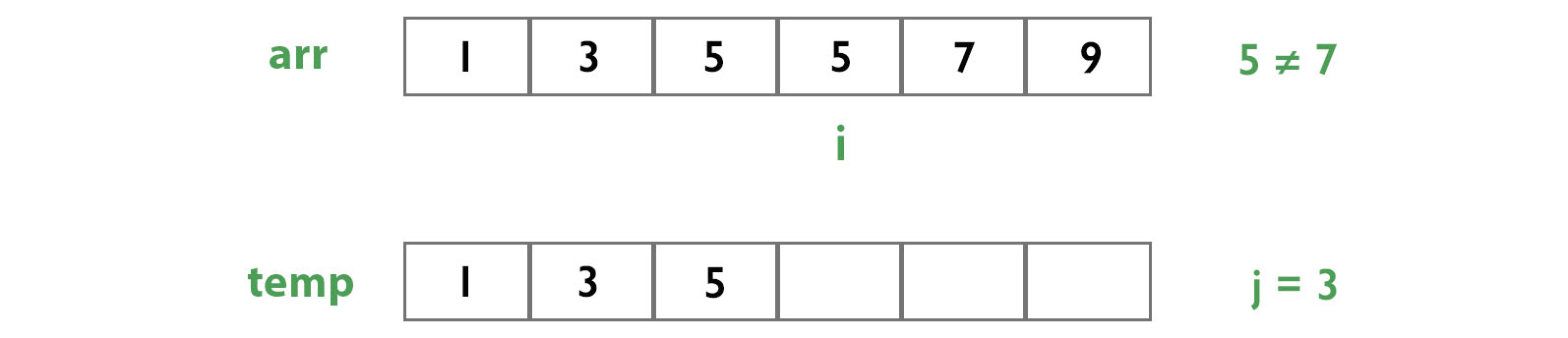7 is not equal to 9. So, 7 will be stored in temp and i will be incremented to point to the index of 9.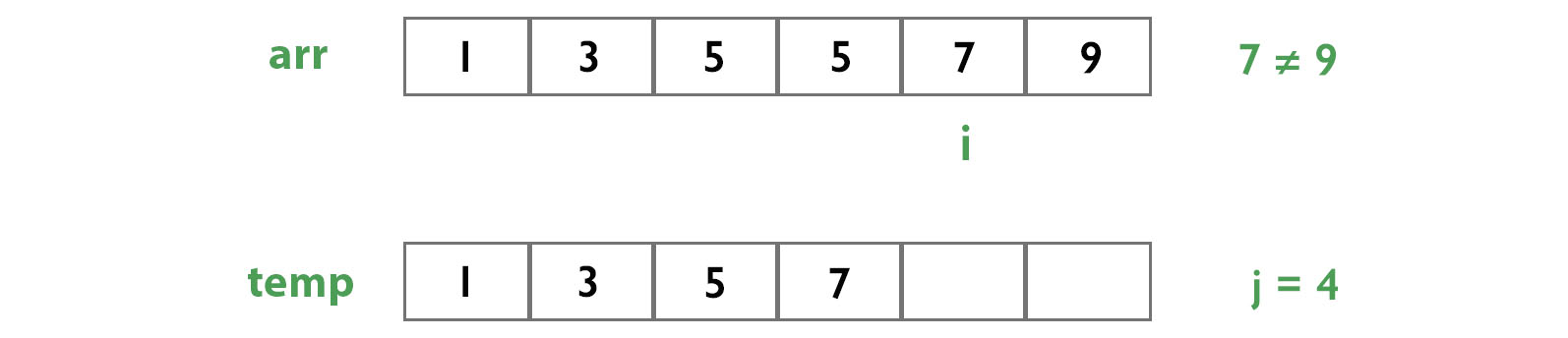At last, we will automatically add 9 to temp.So, the array temp contains all non-duplicate elements of arr. Copy the j elements of temp to arr and print them.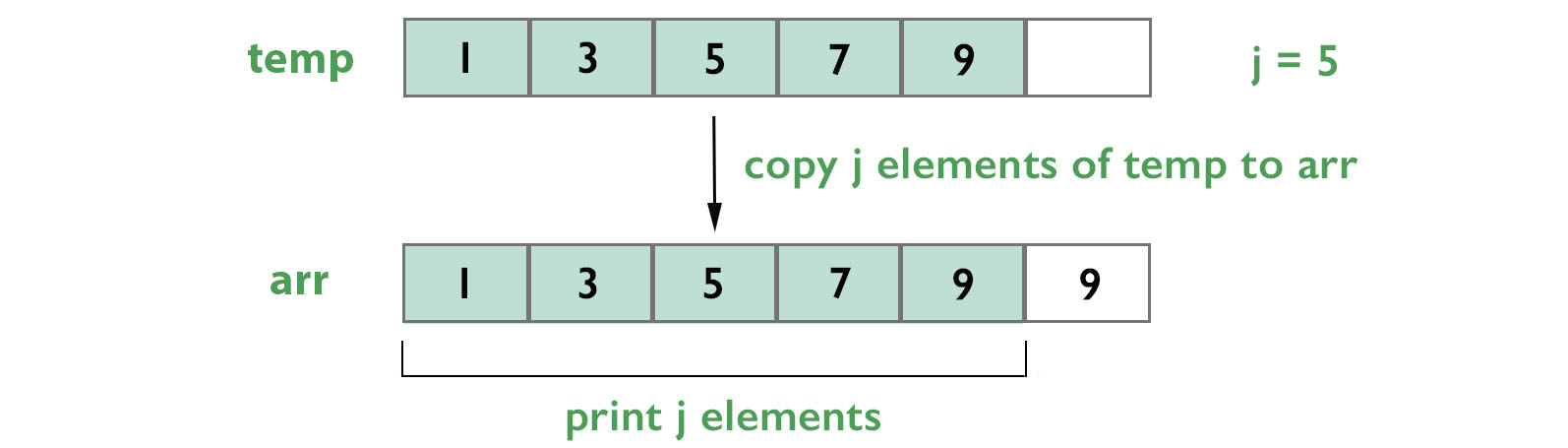### Pseudo Code

j = 0

// traverse elements of arr
for i=0 to n-2
// if ith element is not equal to (i+1)th element of arr, then store ith value in temp
if arr[i] != arr[i+1]
temp[j] = arr[i]
j += 1

// store last value of arr in temp
temp[j] = arr[n-1]
j += 1

// copy elements of temp to first j elements of arr
for i=0 to j-1
arr[i] = temp[i]

// print first j elements of array arr
for i=0 to j-1
print arr[i]

• C/C++
• Python
• Java
#include <stdio.h>

// function for removing duplicates
void removeDuplicate(int arr[], int n)
{
int j = 0;
int temp[n];

// traverse elements of arr.
for (int i = 0; i < n-1; i++)
{
// if ith element is not equal to (i+1)th element of arr, then store ith value in temp
if (arr[i] != arr[i+1])
{
temp[j] = arr[i];
j++;
}
}

// store last value of arr in temp
temp[j++] = arr[n-1];

// make array arr equal to temp
for (int i = 0; i < j; i++)
{
arr[i] = temp[i];
}

// print final array arr
for (int i = 0; i < j; i++)
{
printf("%d ", arr[i]);
}

}

int main()
{
int arr[] = {1, 3, 5, 5, 7, 9};
int n = sizeof(arr) / sizeof(arr);

// calling function when number of elements in array is greater than 1
if (n > 1)
{
removeDuplicate(arr, n);
}
return 0;
}

# function for removing duplicates
def removeDuplicate(arr, n):
j = 0
temp = list(range(n))

# traverse elements of arr.
for i in range(0, n-1):
# if ith element is not equal to (i+1)th element of arr, then store ith value in temp
if (arr[i] != arr[i+1]):
temp[j] = arr[i]
j = j+1

# store last value of arr in temp
temp[j] = arr[n-1]
j = j+1

# copy elements of temp to first j elements of arr
for i in range(0, j):
arr[i] = temp[i]

# print final array arr
for i in range(0, j):
print("%d"%(arr[i]), end = " ")

arr = [1, 3, 5, 5, 7, 9]
n = len(arr)
#calling function when number of elements in array is greater than 1
if (n > 1):
removeDuplicate(arr, n)

class Duplicate
{
// function for removing duplicates
public static void removeDuplicate(int arr[], int n)
{
int j = 0;
int[] temp = new int[n];

// traverse elements of arr.
for (int i = 0; i < n-1; i++)
{
// if ith element is not equal to (i+1)th element of arr, then store ith value in temp
if (arr[i] != arr[i+1])
{
temp[j] = arr[i];
j++;
}
}

// store last value of arr in temp
temp[j++] = arr[n-1];

// copy elements of temp to first j elements of arr
for (int i = 0; i < j; i++)
{
arr[i] = temp[i];
}

// print final array arr
for (int i = 0; i < j; i++)
{
System.out.print(arr[i]+" ");
}

}

public static void main (String[] args)
{
int arr[] = {1, 3, 5, 5, 7, 9};
int n = arr.length;

// calling function when number of elements in array is greater than 1
if (n > 1)
{
removeDuplicate(arr, n);
}
}
}


Output

1 3 5 7 9

### Time Complexity

The time complexity is O(N).

## Method 2 – Without using extra space

This method is the same as the previous one, but we will not make a seperate array (temp) like in the previous method. Instead, we will store our final resultant elements in the same array (arr) instead of temp.

### Pseudo Code

j = 0

// traverse elements of arr
for i=0 to n-2
// if ith element is not equal to (i+1)th element of arr, then store ith value in arr[j]
if arr[i] != arr[i+1]
arr[j] = arr[i]
j += 1

// store last value of arr in temp
arr[j] = arr[n-1]
j += 1

// print first j elements of array arr
for i=0 to j-1
print arr[i]

• C/C++
• Python
• Java
#include <stdio.h>

// function for removing duplicates
void removeDuplicate(int arr[], int n)
{
int j = 0;

// traverse elements of arr
for (int i = 0; i < n-1; i++)
{
// if ith element is not equal to (i+1)th element, then store ith value in arr[j]
if (arr[i] != arr[i+1])
{
arr[j] = arr[i];
j++;
}
}

// store last value of arr in arr[j]
arr[j++] = arr[n-1];

// print first j elements of array arr
for (int i = 0; i < j; i++)
{
printf("%d ", arr[i]);
}

}

int main()
{
int arr[] = {1, 3, 5, 5, 7, 9};
int n = sizeof(arr) / sizeof(arr);
// calling function when number of elements in array is greater than 1
if (n > 1)
{
removeDuplicate(arr, n);
}
return 0;
}

# function for removing duplicates
def removeDuplicate(arr, n):
j = 0

# traverse elements of arr
for i in range(0, n-1):
# if ith element is not equal to (i+1)th element, then store ith value in arr[j]
if (arr[i] != arr[i+1]):
arr[j] = arr[i]
j = j+1

# store last value of arr in arr[j]
arr[j] = arr[n-1]
j = j+1

# print first j elements of array arr
for i in range(0, j):
print("%d"%(arr[i]), end = " ")

arr = [1, 3, 5, 5, 7, 9]
n = len(arr)
# calling function when number of elements in array is greater than 1
if (n > 1):
removeDuplicate(arr, n)

class Duplicate
{

// function for removing duplicates
public static void removeDuplicate(int arr[], int n) {

int j = 0;

// traverse elements of arr
for (int i = 0; i < n-1; i++)
{
// if ith element is not equal to (i+1)th element, then store ith value in arr[j]
if (arr[i] != arr[i+1])
{
arr[j] = arr[i];
j++;
}
}

// store last value of arr in arr[j]
arr[j++] = arr[n-1];

// print first j elements of array arr
for (int i = 0; i < j; i++)
{
System.out.print(arr[i]+" ");
}

}

public static void main (String[] args)
{
int arr[] = {1, 3, 5, 5, 7, 9};
int n = arr.length;
// calling function when number of elements in array is greater than 1
if (n > 1)
{
removeDuplicate(arr, n);
}
}
}


### Time Complexity

The time complexity is O(N).

Liked the post?
0 COMMENT